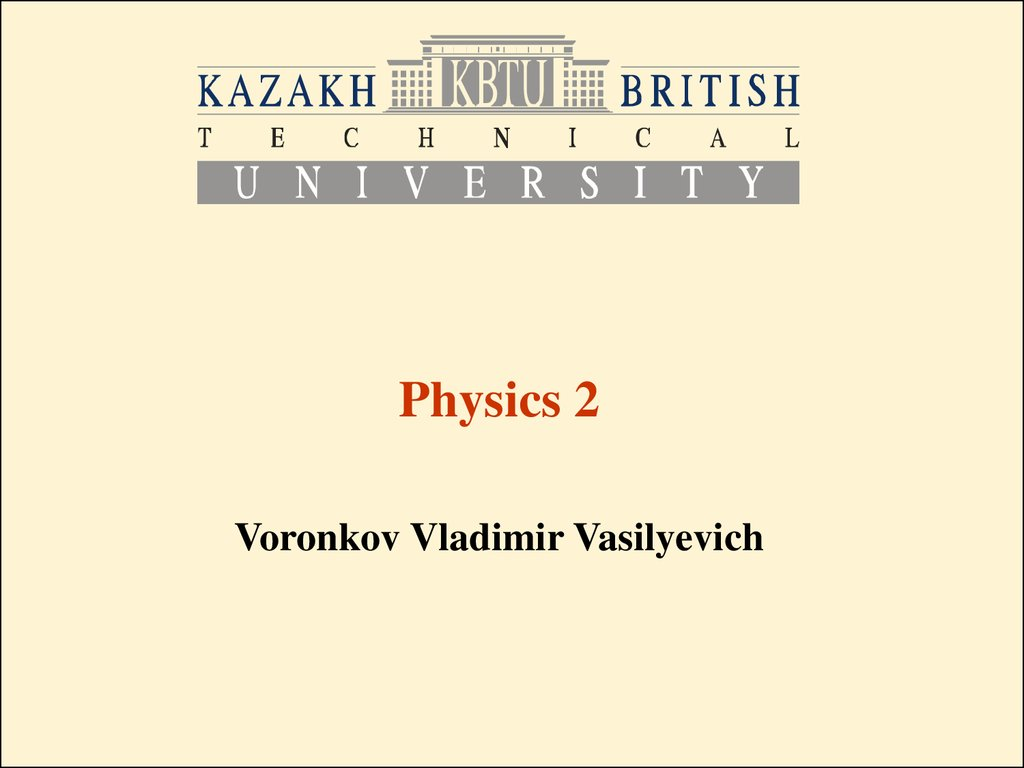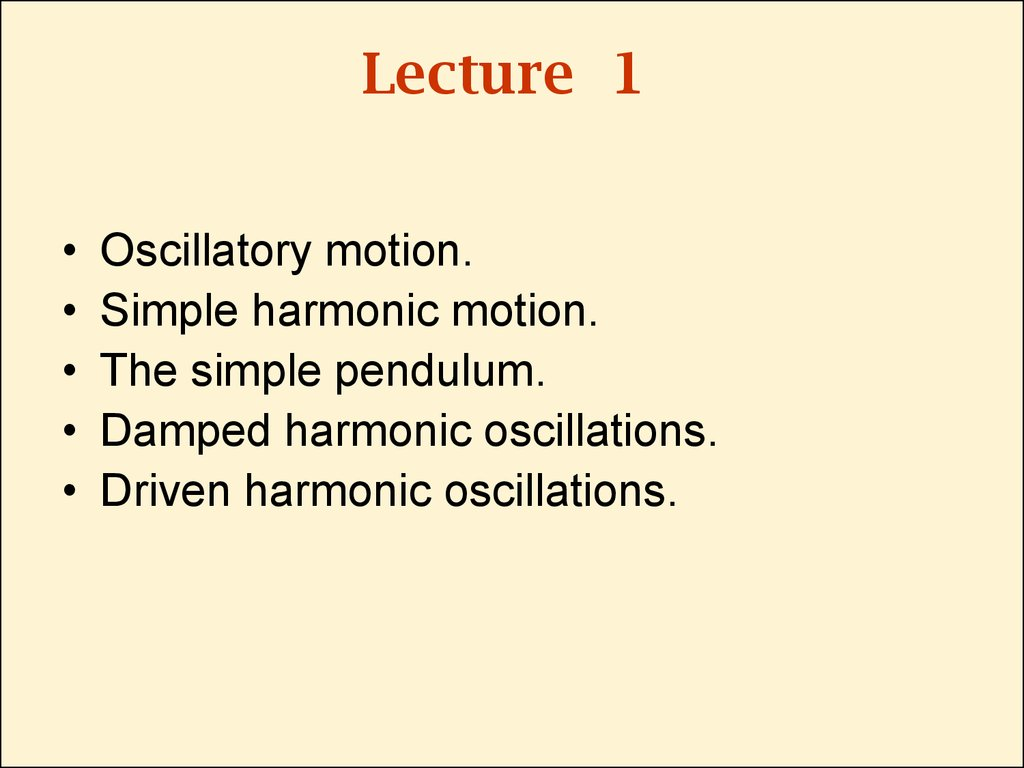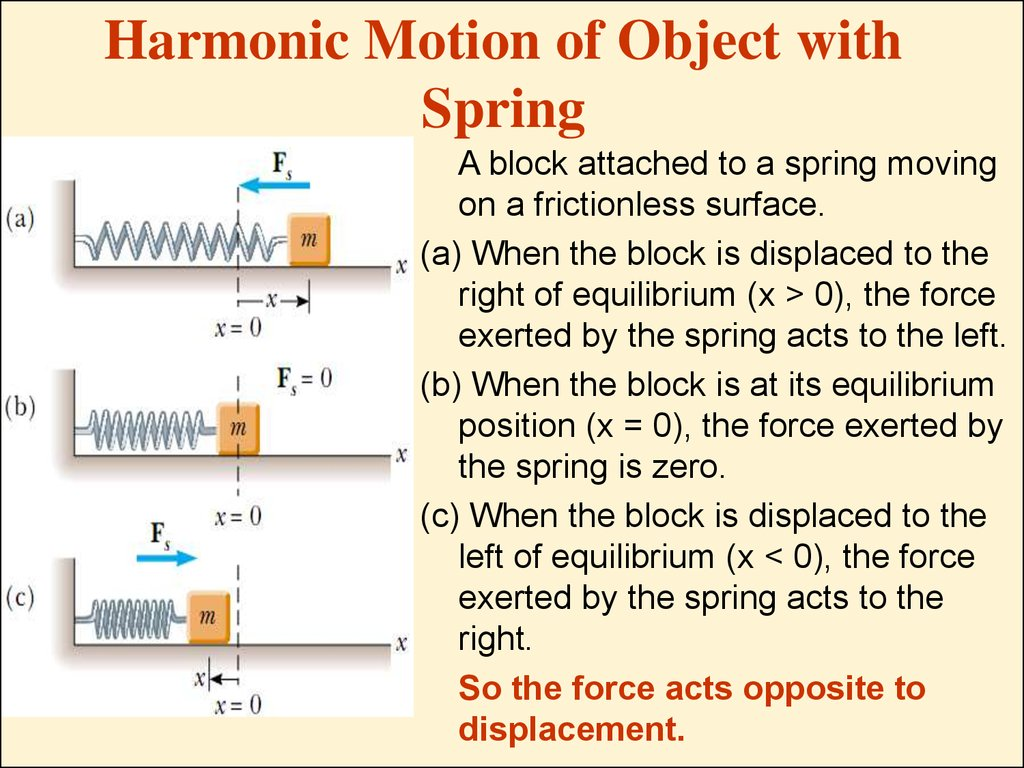# Oscillatory motion. Simple harmonic motion. The simple pendulum. Damped harmonic oscillations. (Lecture 1)

## 1.

Physics 2
Voronkov Vladimir Vasilyevich

## 2. Lecture 1

Oscillatory motion.
Simple harmonic motion.
The simple pendulum.
Damped harmonic oscillations.
Driven harmonic oscillations.

## 3. Harmonic Motion of Object with Spring

A block attached to a spring moving
on a frictionless surface.
(a) When the block is displaced to the
right of equilibrium (x > 0), the force
exerted by the spring acts to the left.
(b) When the block is at its equilibrium
position (x = 0), the force exerted by
the spring is zero.
(c) When the block is displaced to the
left of equilibrium (x < 0), the force
exerted by the spring acts to the
right.
So the force acts opposite to
displacement.

## 4.

• x is displacement from equilibrium position.
• Restoring force is given by Hook’s law:
• Then we can obtain the acceleration:
• That is, the acceleration is proportional to the
position of the block, and its direction is
opposite the direction of the displacement
from equilibrium.

## 5. Simple Harmonic Motion

• An object moves with simple harmonic
motion whenever its acceleration is
proportional to its position and is oppositely
directed to the displacement from
equilibrium.

## 6.

Mathematical Representation
of Simple Harmonic Motion
• So the equation for harmonic motion is:
• We can denote angular frequency as:
• Then:
• Solution for this equation is:

## 7.

• A=const is the amplitude of the motion
• w=const is the angular frequency of the
motion
• f=const is the phase constant
• wt+f is the phase of the motion
• T=const is the period of oscillations:

## 8.

• The inverse of the period is the frequency f of
the oscillations:

## 9.

• Then the velocity and the acceleration of a body
in simple harmonic motion are:

## 10.

• Position vs time
• Velocity vs time
At any specified time the
velocity is 90° out of phase
with the position.
• Acceleration vs time
At any specified time the
acceleration is 180° out of
phase with the position.

## 11. Energy of the Simple Harmonic Oscillator

• Assuming that:
– no friction
– the spring is massless
• Then the kinetic energy of system spring-body
corresponds only to that of the body:
• The potential energy in the spring is:

## 12.

• The total mechanical energy of simple
harmonic oscillator is:
• That is, the total mechanical energy of a simple
harmonic oscillator is a constant of the motion
and is proportional to the square of the
amplitude.

## 13. Simple Pendulum

• Simple pendulum consists of a
particle-like bob of mass m
suspended by a light string of
length L that is xed at the
upper end.
• The motion occurs in the
vertical plane and is driven by
the gravitational force.
• When Q is small, a simple
pendulum oscillates in simple
harmonic motion about the
equilibrium position Q = 0. The
restoring force is -mgsinQ, the
component of the gravitational
force tangent to the arc.

## 14.

• The Newton’s second law in tangential
direction:
• For small values of Q :
• Solution for this equation is:

## 15.

• The period and frequency of a simple
pendulum depend only on the length of the
string and the acceleration due to gravity.
• The simple pendulum can be used as a
timekeeper because its period depends
only on its length and the local value of g.

## 16. Physical Pendulum

If a hanging object oscillates
about a xed axis that does
not pass through its center of
mass and the object cannot
be approximated as a point
mass, we cannot treat the
system as a simple pendulum.
In this case the system is
called a physical pendulum.

## 17.

• Applying the rotational form of the second
Newton’s law:
• The solution is:
• The period is

## 18. Damped Harmonic Oscillations

• In many real systems, nonconservative
forces, such as friction, retard the motion.
Consequently, the mechanical energy of
the system diminishes in time, and the
motion is damped. The retarding force can
be expressed as R=-bv (b=const is the
damping coefficient) and the restoring force
of the system is -kx then:

## 19.

• The solution for small b is
• When the retarding force is small, the
oscillatory character of the motion is
preserved but the amplitude decreases
in time, with the result that the motion
ultimately ceases.

## 20.

• The angular frequency can be expressed
through w0=(k/m)1/2 – the natural frequency of
the system (the undamped oscillator):

## 21.

(a) underdamped oscillator:
Rmax=bVmax<kA. System
oscillates with damping
amplitude
(b) critically damped oscillator:
when b has critical value bc=
2mw0 . System does not
oscillate, just returns to the
equilibrium position.
(c) overdamped oscillator:
Rmax=bVmax>kA and
b/(2m)>w0 . System does not
oscillate, just returns to the
equilibrium position.

## 22. Driven Harmonic Oscillations

• A driven (or forced) oscillator is a damped
oscillator under the influence of an external
periodical force F(t)=F0sin(wt). The second
Newton’s law for forced oscillator is:
• The solution of this equation is:

## 23.

• The forced oscillator vibrates at the frequency
of the driving force
• The amplitude of the oscillator is constant
for a given driving force.
• For small damping, the amplitude is large
when the frequency of the driving force is
near the natural frequency of oscillation, or
when w w0.
• The dramatic increase in amplitude near the
natural frequency is called resonance, and
the natural frequency w0 is also called the
resonance frequency of the system.

## 24. Resonance

• So resonance happens when the driving force
frequency is close to the natural frequency of
the system: w w0. At resonance the amplitude
of the driven oscillations is the largest.
• In fact, if there were no damping (b = 0), the
amplitude would become infinite when w=w0.
This is not a realistic physical situation, because
it corresponds to the spring being stretched to
infinite length. A real spring will snap rather
than accept an infinite stretch; in other words,
some for of damping will ultimately occur, But
it does illustrate that, at resonance, the response
of a harmonic system to a driving force can be
catastrophically large.

## 25. Units in Si

spring constant
damping coefficient
phase
angular frequency
frequency
period
k
b
f
w
f
T
N/m=kg/s2
kg/s
rad (or degrees)
rad/s
1/s
s